# 10 most difficult puzzles in the world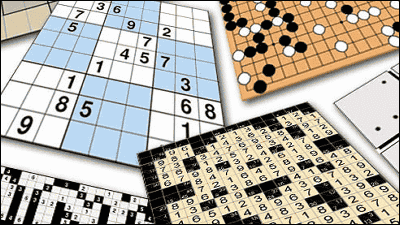For a short timepuzzleIs the best. A puzzle enthusiast and a person not so often talk about puzzles in smartphones. It is a puzzle loved all over the world regardless of age and sex, but what kind of thing is the most difficult level?

10 most difficult puzzles in the world
http://www.conceptispuzzles.com/ja/index.aspx?uri=info/article/424

◆ 01. Kanpure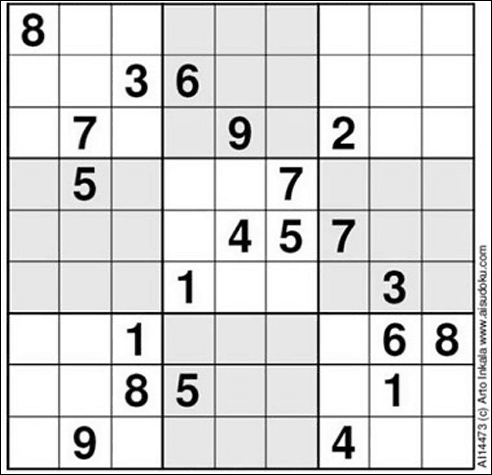This is the most difficult question of "numberplace" so-called "Nanpure". Sudoku is a puzzle called "Put numbers from 1 to 9 in 3 × 3 blocks surrounded by vertical and horizontal columns and bold lines so that the same numbers are not placed in multiple blocks". Theoretically, the minimum number of preset numbers seems to be 17, but this problem is somewhat larger than 21. However, it is a ridiculous setting that the numbers placed in the left and right columns are only 8. Usually the level of Sudoku is displayed in 1 to 5 stages, this puzzle is amazingLevel 11. no longerEven hard to imagine the difficultyI do not understand the situation.

◆ 02. The riddle

There are three gods A, B, C. Three people have the name "true" "fake" "mood shop". True saying is always right, fake always lies, and the mood shops say the right things to the whim and lie. Please repeat the question answered with YES or NO three times and find the identity of A, B, C. However, one question will be directed to one God. Although God understands the meaning of the question, the answer is returned with their own words "da" or "ja". "Da" and "Ja" are words equivalent to "YES" and "NO", but you do not know which is meaning which.

The problem here to be told "The riddle that is the hardest in history". It was invented by American philosopher / logicologist George Boolos. By the way riddles are handled as one of the puzzles in "Logic Puzzle (logical puzzle)". The place where God answers only in "da" "ja" is a miso of this problem. We are improving the difficulty level dramatically. Do philosophers think only about such logic problems ...?

Below is a paper on Mr. Boolos's process of making this riddle, the relation between logic and language is considered.

The Hardest Logic Puzzle Ever 'Made Even Harder

◆ 03. Sam Nunpre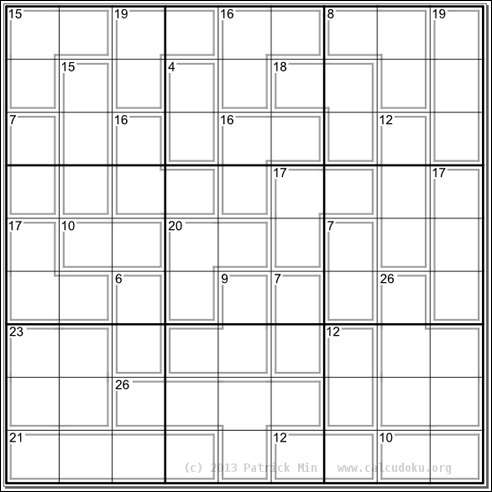This is the most difficult question of Nanpure's derivative puzzle 'Samnappl'. Samnonpre said, "While making the total of the numbers entering the area divided by the frame equal to the number written in the upper left of the area, 3 × 3 blocks separated by vertical columns, horizontal rows, bold lines Put a number from 1 to 9 in each one "is a puzzle. Of course the rule of not including the same number in 3 × 3 blocks is the same as Sumire. There are no initial numbers placed on this problem ...... It seems to only break through from where there are 1 - 3 or 3 - 1 street within the frame with the number 4 on the top left.

◆ 04. Bongguard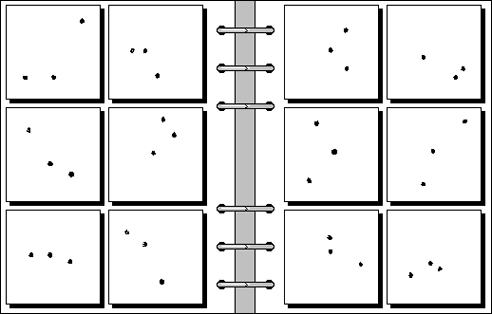This is the most challenging puzzle called "bongard". Bongard is a puzzle invented by Russian computer scientist Mikhail Moiseevich Bongard who looks for patterns and regularity in the six boxes on the left and hints that the six boxes on the right do not have the same pattern or regularity It is given. Speaking of common points between the left and right boxes, I can only think of "there are three points". Even if there is regularity in the left and right boxes in the first place it is impossible to distinguish. I doubt even whether I can understand the correct answer.

◆ 05. Inspection Log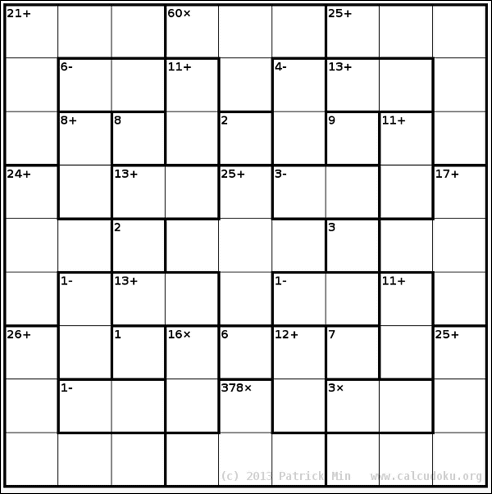This is the most difficult question of "Inspection Log". Inspection is said to have been devised by Mr. Tetsuya Miyamoto in a puzzle "put numeric figures from 1 to the number of columns in the vertical and horizontal columns and use the arithmetic operator on the upper left to make the number on the upper left". I will. This problem is a puzzle site "Online Calcudoku"It was said that the correct answer rate was 9.6%. I am surprised that one in 10 people could solve it.

Online Calcudoku
http://www.calcudoku.org/ja

◆ 06. Ponder this Puzzle

Design a storage system that encodes 24 information bits on 8 disks of 4 bits each. The conditions are as follows.
(1) Join 8 * 4 bits to 32 bit number (pick nibble from each disk). The variable f from 24 bits to 32 bits can be found using only five operations. Each bit is set on a variable-length integer as a set {+, -, *, /,%, & amp ;, | ~} (addition, subtraction, multiplication, integer division, modulo, bitwise logical product; bitwise OR, Bitwise negation operator) is missing. In other words, assuming that each operation is done in 1 nanosecond, the variable is calculated in 5 ns.
(2) It is possible to recover the first 24 bits even if two of 8 disks crash and it is not readable and 2 nibbles are lost.

IBM Reserch has been puzzling monthly on "Ponder this", but this question is that the call to be the most challenging is issued in April 2009. This is also a pattern that it is difficult to understand the meaning of the problem in the first place, the difficulty of the problem itself is not transmitted. Programmers are impressed with building such a system with such a proposition.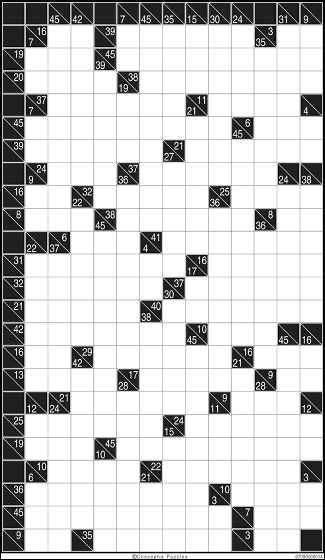This is "the addition puzzle" is the most difficult question "Kakuro". The addition puzzle is a logic puzzle in which Sudoku and Crosswords are mixed. "As the number in the triangular square starts from there, it becomes the sum of the numbers entering each of the vertical and horizontal blocks, A number from 1 to 9 will be inserted. " It is an interesting rule puzzle, but there is a sense of intimidation that does not make me think "to solve well!

◆ 08. Martin Gardner's Mathematical Puzzle
The Colossal Book of Short Puzzles And Problems
http://www.amazon.co.jp/dp/0393061140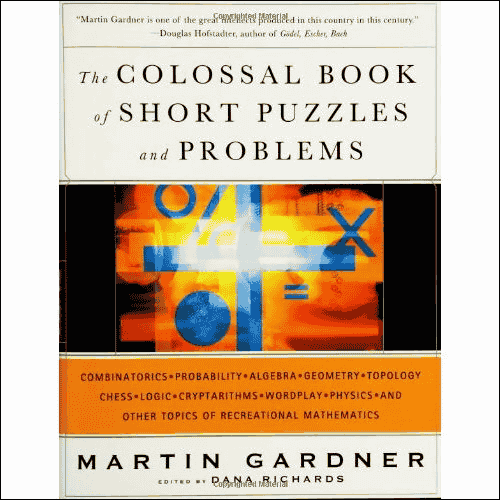American mathematician Martin Gardner is a popular person in a wide range to explain the latest mathematics in an easy-to-understand manner. The puzzles devised by Dr. Gardner are recorded in "The Colossal Book of Short Puzzles And Problems"is. This book contains more than 300 questions from simple problems to super difficult challenges, and it has become popular among mathematicians as being able to enjoy according to your mathematical skills.

◆ 09. Go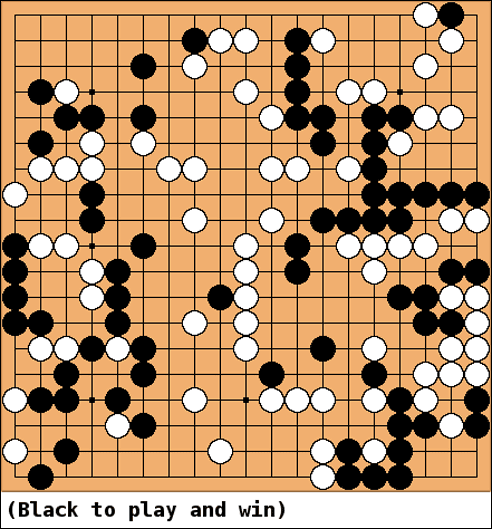This is the most difficult question of "go".A goWhat is Tashi Shogi 's Go version? By the way, this problem, even the experts of Go will take 1000 hours to answer. If it is 1000 hours, it seems that beginner can be intermediate level ... ....

◆ 10. Mosaic art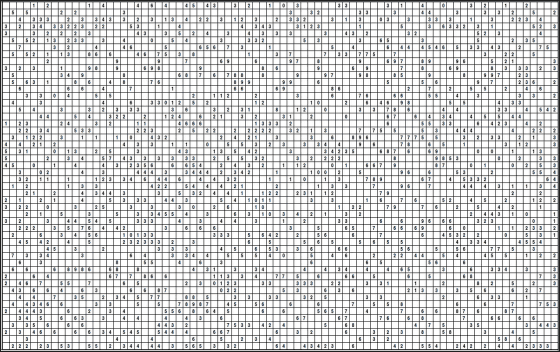Mosaic art is a rules puzzle similar to Minesweeper. The numerical value of the trout shows the number of squares to be painted in the 3 × 3 range (within 9 squares) centering on that square, and the illustration floats up when all the squares are painted out. The following is the most challenging mosaic art that Trevor Truran invented. As to what will happen when answering,click hereIt is possible to see it, its form is just art.

The common thing to all puzzles is "The rules themselves are simple, but the depth of the end is endless"something like. "It's as hard as simple things" is the perfection that makes you realize that it is a truth that applies to everything, not just puzzles.

in Science,   Game,   Art, Posted by darkhorse_log